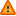FAQ/equalwts - CBU statistics WikiYou should trust both wikis because the password could be read by the particular administrators.
The harmonic mean may also be used to weight unequal group sample sizes to compute Type III (unweighted) sum of squares in an analysis of variance. To see this unweighting charatceristic suppose we have three sample sizes 3, 6 and 9 which we wish to average. The harmonic mean for these three sample sizes is $$\frac{\mbox{3x3 + 1.5x6 + 9x1}}{\mbox{3 + 1.5 + 1}}$$ = 4.9. Each sample size contributes the same amount to the numerator, 9, which is equal to the highest sample size. In this way smaller sample sizes contribute more to the computation of the average sample size than using the arithmetic mean. Indeed, the harmonic mean is always less than or equal to the arithmetic mean.
More generally for k group sample sizes, $$n_text{1}, ..., n_text{k}$$ the harmonic mean may be expressed as $$\frac{k n_text{k}}{n_text{k} \sum_text{j=1}^text{k} \frac{1}{n_text{j}}}$$. This expression reduces to the more familiar form after dividing through by $$n_text{k}$$.